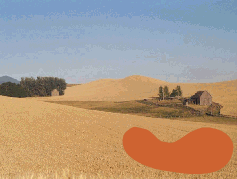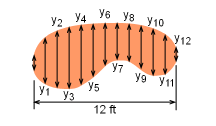Ch 8. Integration Techniques Multimedia Engineering Math Integration byParts Trig. IntegralsTrig. Subst. Fractions& Substitutions ApproximateIntegration
 Chapter 1. Limits 2. Derivatives I 3. Derivatives II 4. Mean Value 5. Curve Sketching 6. Integrals 7. Inverse Functions 8. Integration Tech. 9. Integrate App. 10. Parametric Eqs. 11. Polar Coord. 12. Series Appendix Basic Math Units Search eBooks Dynamics Fluids Math Mechanics Statics Thermodynamics Author(s): Hengzhong Wen Chean Chin Ngo Meirong Huang Kurt Gramoll ©Kurt GramollMATHEMATICS - CASE STUDY

IntroductionUFO Attack the Wheat Field

One early morning, Jack discovered a mysterious large kidney-shaped formation in his wheat field. He was surprised and realized that he needed to estimate his wheat loss in that region.

What is known:

Jack measured at 1-foot intervals as indicated in the following figure.y0 1.5 y5 4.4 y10 3.8 y1 3.5 y6 3.5 y11 3.5 y2 4.8 y7 2.4 y12 1.5 y3 5.3 y8 3.1 y4 5 y9 3.6

Questions

Determine the area of the kidney-shaped formation using the Midpoint Rule, Trapezoidal Rule, and Simpson's Rule.

Approach

• Midpoint Rule
Area = Δx(y0 + y1 + y2 + ... + y12)
• Trapezoidal Rule
Area = Δx/2(y0 + 2y1 +2 y2 + ... + 2y11 + y12)
• Simpson's Rule
Area = h/3(y0 + 4y1 +2 y2 + ... + 4y11 + y12)